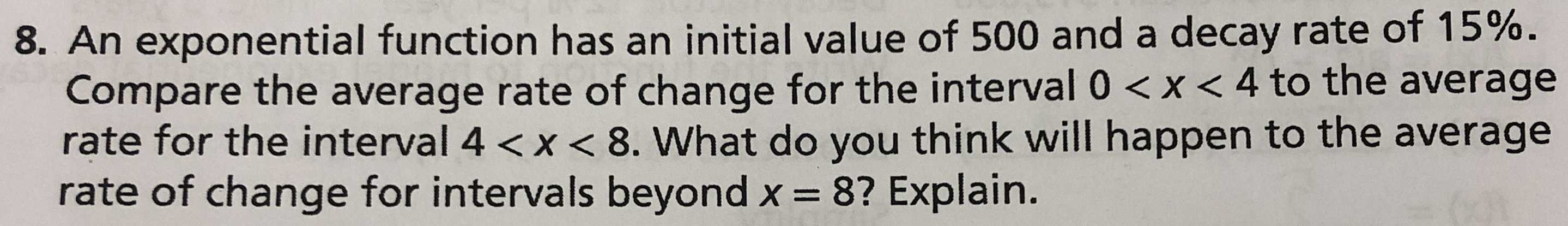### ¿Todavía tienes preguntas de matemáticas?

Pregunte a nuestros tutores expertos
Algebra
Pregunta8. An exponential function has an initial value of $$500$$ and a decay rate of $$15 \%$$ .  Compare the average rate of change for the interval $$0 < x < 4$$ to the average  rate for the interval $$4 < x < 8$$ . What do you think will happen to the average  rate of change for intervals beyond $$x = 8$$ ? Explain.